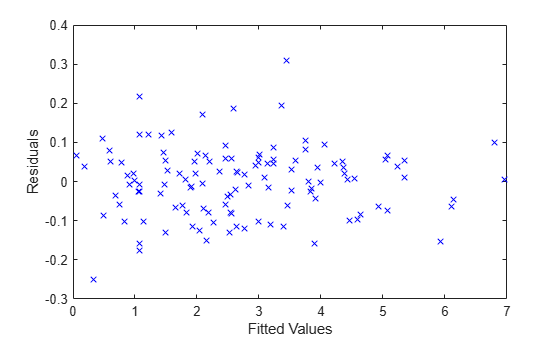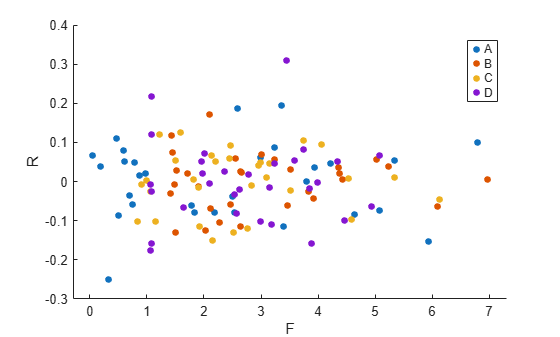# fitted

Class: LinearMixedModel

Fitted responses from a linear mixed-effects model

## Syntax

``yfit = fitted(lme)``
``yfit = fitted(lme,Name,Value)``

## Description

````yfit = fitted(lme)` returns the fitted conditional response from the linear mixed-effects model `lme`.```

example

````yfit = fitted(lme,Name,Value)` returns the fitted response from the linear mixed-effects model `lme` with additional options specified by one or more `Name,Value` pair arguments.For example, you can specify if you want to compute the fitted marginal response.```

## Input Arguments

expand all

Linear mixed-effects model, specified as a `LinearMixedModel` object constructed using `fitlme` or `fitlmematrix`.

### Name-Value Pair Arguments

Specify optional comma-separated pairs of `Name,Value` arguments. `Name` is the argument name and `Value` is the corresponding value. `Name` must appear inside quotes. You can specify several name and value pair arguments in any order as `Name1,Value1,...,NameN,ValueN`.

Indicator for conditional response, specified as the comma-separated pair consisting of `'Conditional'` and either of the following.

 `true` Contribution from both fixed effects and random effects (conditional) `false` Contribution from only fixed effects (marginal)

Example: `'Conditional',false`

Data Types: `logical`

## Output Arguments

expand all

Fitted response values, returned as an n-by-1 vector, where n is the number of observations.

## Examples

expand all

`load flu`

The `flu` dataset array has a `Date` variable, and 10 variables containing estimated influenza rates (in 9 different regions, estimated from Google® searches, plus a nationwide estimate from the Center for Disease Control and Prevention, CDC).

To fit a linear-mixed effects model, your data must be in a properly formatted dataset array. To fit a linear mixed-effects model with the influenza rates as the responses and region as the predictor variable, combine the nine columns corresponding to the regions into an array. The new dataset array, `flu2`, must have the response variable, `FluRate`, the nominal variable, `Region`, that shows which region each estimate is from, and the grouping variable `Date`.

```flu2 = stack(flu,2:10,'NewDataVarName','FluRate','IndVarName','Region'); flu2.Date = nominal(flu2.Date);```

Fit a linear mixed-effects model with fixed effects for region and a random intercept that varies by `Date`.

Region is a categorical variable. You can specify the contrasts for categorical variables using the `DummyVarCoding` name-value pair argument when fitting the model. When you do not specify the contrasts, `fitlme` uses the `'reference'` contrast by default. Because the model has an intercept, `fitlme` takes the first region, `NE`, as the reference and creates eight dummy variables representing the other eight regions. For example, $I\left[MidAtl\right]$ is the dummy variable representing the region `MidAtl`. For details, see Dummy Variables.

The corresponding model is

`$\begin{array}{l}{y}_{im}={\beta }_{0}+{\beta }_{1}I{\left[MidAtl\right]}_{i}+{\beta }_{2}I{\left[ENCentral\right]}_{i}+{\beta }_{3}I{\left[WNCentral\right]}_{i}+{\beta }_{4}I{\left[SAtl\right]}_{i}\\ \phantom{\rule{1em}{0ex}}\phantom{\rule{1em}{0ex}}+{\beta }_{5}I{\left[ESCentral\right]}_{i}+{\beta }_{6}I{\left[WSCentral\right]}_{i}+{\beta }_{7}I{\left[Mtn\right]}_{i}+{\beta }_{8}I{\left[Pac\right]}_{i}+{b}_{0m}+{\epsilon }_{im},\phantom{\rule{1em}{0ex}}m=1,2,...,52,\end{array}$`

where ${y}_{im}$ is the observation $i$ for level $m$ of grouping variable `Date`, ${\beta }_{j}$, $j$ = 0, 1, ..., 8, are the fixed-effects coefficients, with ${\beta }_{0}$ being the coefficient for region `NE`. ${b}_{0m}$ is the random effect for level $m$ of the grouping variable `Date`, and ${\epsilon }_{im}$ is the observation error for observation $i$. The random effect has the prior distribution, ${b}_{0m}\sim N\left(0,{\sigma }_{b}^{2}\right)$ and the error term has the distribution, ${\epsilon }_{im}\sim N\left(0,{\sigma }^{2}\right)$.

`lme = fitlme(flu2,'FluRate ~ 1 + Region + (1|Date)')`
```lme = Linear mixed-effects model fit by ML Model information: Number of observations 468 Fixed effects coefficients 9 Random effects coefficients 52 Covariance parameters 2 Formula: FluRate ~ 1 + Region + (1 | Date) Model fit statistics: AIC BIC LogLikelihood Deviance 318.71 364.35 -148.36 296.71 Fixed effects coefficients (95% CIs): Name Estimate SE tStat DF {'(Intercept)' } 1.2233 0.096678 12.654 459 {'Region_MidAtl' } 0.010192 0.052221 0.19518 459 {'Region_ENCentral'} 0.051923 0.052221 0.9943 459 {'Region_WNCentral'} 0.23687 0.052221 4.5359 459 {'Region_SAtl' } 0.075481 0.052221 1.4454 459 {'Region_ESCentral'} 0.33917 0.052221 6.495 459 {'Region_WSCentral'} 0.069 0.052221 1.3213 459 {'Region_Mtn' } 0.046673 0.052221 0.89377 459 {'Region_Pac' } -0.16013 0.052221 -3.0665 459 pValue Lower Upper 1.085e-31 1.0334 1.4133 0.84534 -0.092429 0.11281 0.3206 -0.050698 0.15454 7.3324e-06 0.13424 0.33949 0.14902 -0.02714 0.1781 2.1623e-10 0.23655 0.44179 0.18705 -0.033621 0.17162 0.37191 -0.055948 0.14929 0.0022936 -0.26276 -0.057514 Random effects covariance parameters (95% CIs): Group: Date (52 Levels) Name1 Name2 Type Estimate {'(Intercept)'} {'(Intercept)'} {'std'} 0.6443 Lower Upper 0.5297 0.78368 Group: Error Name Estimate Lower Upper {'Res Std'} 0.26627 0.24878 0.285 ```

The $p$-values 7.3324e-06 and 2.1623e-10 respectively show that the fixed effects of the flu rates in regions `WNCentral` and `ESCentral` are significantly different relative to the flu rates in region `NE`.

The confidence limits for the standard deviation of the random-effects term, ${\sigma }_{b}$, do not include 0 (0.5297, 0.78368), which indicates that the random-effects term is significant. You can also test the significance of the random-effects terms using the `compare` method.

The conditional fitted response from the model at a given observation includes contributions from fixed and random effects. For example, the estimated best linear unbiased predictor (BLUP) of the flu rate for region `WNCentral` in week 10/9/2005 is

`$\begin{array}{l}{\underset{}{\overset{ˆ}{y}}}_{WNCentral,10/9/2005}={\underset{}{\overset{ˆ}{\beta }}}_{0}+{\underset{}{\overset{ˆ}{\beta }}}_{3}I\left[WNCentral\right]+{\underset{}{\overset{ˆ}{b}}}_{10/9/2005}\\ \phantom{\rule{1em}{0ex}}\phantom{\rule{1em}{0ex}}\phantom{\rule{1em}{0ex}}\phantom{\rule{1em}{0ex}}\phantom{\rule{1em}{0ex}}\phantom{\rule{1em}{0ex}}\phantom{\rule{1em}{0ex}}\phantom{\rule{1em}{0ex}}=1.2233+0.23687-0.1718\\ \phantom{\rule{1em}{0ex}}\phantom{\rule{1em}{0ex}}\phantom{\rule{1em}{0ex}}\phantom{\rule{1em}{0ex}}\phantom{\rule{1em}{0ex}}\phantom{\rule{1em}{0ex}}\phantom{\rule{1em}{0ex}}\phantom{\rule{1em}{0ex}}=1.28837.\end{array}$`

This is the fitted conditional response, since it includes contributions to the estimate from both the fixed and random effects. You can compute this value as follows.

```beta = fixedEffects(lme); [~,~,STATS] = randomEffects(lme); % Compute the random-effects statistics (STATS) STATS.Level = nominal(STATS.Level); y_hat = beta(1) + beta(4) + STATS.Estimate(STATS.Level=='10/9/2005')```
```y_hat = 1.2884 ```

In the previous calculation, `beta(1)` corresponds to the estimate for ${\beta }_{0}$ and `beta(4)` corresponds to the estimate for ${\beta }_{3}$. You can simply display the fitted value using the `fitted` method.

```F = fitted(lme); F(flu2.Date == '10/9/2005' & flu2.Region == 'WNCentral')```
```ans = 1.2884 ```

The estimated marginal response for region `WNCentral` in week 10/9/2005 is

`$\begin{array}{l}{\underset{}{\overset{ˆ}{y}}}_{WNCentral,10/9/2005}^{\left(marginal\right)}={\underset{}{\overset{ˆ}{\beta }}}_{0}+{\underset{}{\overset{ˆ}{\beta }}}_{3}I\left[WNCentral\right]\\ \phantom{\rule{1em}{0ex}}\phantom{\rule{1em}{0ex}}\phantom{\rule{1em}{0ex}}\phantom{\rule{1em}{0ex}}\phantom{\rule{1em}{0ex}}\phantom{\rule{1em}{0ex}}\phantom{\rule{1em}{0ex}}\phantom{\rule{1em}{0ex}}=1.2233+0.23687\\ \phantom{\rule{1em}{0ex}}\phantom{\rule{1em}{0ex}}\phantom{\rule{1em}{0ex}}\phantom{\rule{1em}{0ex}}\phantom{\rule{1em}{0ex}}\phantom{\rule{1em}{0ex}}\phantom{\rule{1em}{0ex}}\phantom{\rule{1em}{0ex}}=1.46017.\end{array}$`

Compute the fitted marginal response.

```F = fitted(lme,'Conditional',false); F(flu2.Date == '10/9/2005' & flu2.Region == 'WNCentral')```
```ans = 1.4602 ```

`load(fullfile(matlabroot,'examples','stats','weight.mat'));`

`weight` contains data from a longitudinal study, where 20 subjects are randomly assigned to 4 exercise programs, and their weight loss is recorded over six 2-week time periods. This is simulated data.

Store the data in a table. Define `Subject` and `Program` as categorical variables.

```tbl = table(InitialWeight,Program,Subject,Week,y); tbl.Subject = nominal(tbl.Subject); tbl.Program = nominal(tbl.Program);```

Fit a linear mixed-effects model where the initial weight, type of program, week, and the interaction between the week and type of program are the fixed effects. The intercept and week vary by subject.

`lme = fitlme(tbl,'y ~ InitialWeight + Program*Week + (Week|Subject)');`

Compute the fitted values and raw residuals.

```F = fitted(lme); R = residuals(lme);```

Plot the residuals versus the fitted values.

```plot(F,R,'bx') xlabel('Fitted Values') ylabel('Residuals')```Now, plot the residuals versus the fitted values, grouped by program.

```figure() gscatter(F,R,Program)```